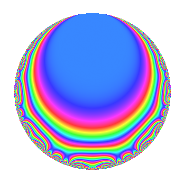Properties

 Label 4000.1.h.bLevel 4000 Weight 1 Character orbit 4000.h Analytic conductor 1.996 Analytic rank 0 Dimension 4 Projective image $$A_{5}$$ CM/RM No Inner twists 2

Related objects

Newspace parameters

 Level: $$N$$ = $$4000 = 2^{5} \cdot 5^{3}$$ Weight: $$k$$ = $$1$$ Character orbit: $$[\chi]$$ = 4000.h (of order $$2$$ and degree $$1$$)

Newform invariants

 Self dual: No Analytic conductor: $$1.99626005053$$ Analytic rank: $$0$$ Dimension: $$4$$ Coefficient field: $$\Q(i, \sqrt{5})$$ Coefficient ring: $$\Z[a_1, \ldots, a_{11}]$$ Coefficient ring index: $$1$$ Projective image $$A_{5}$$ Projective field Galois closure of 5.1.1000000.2

$q$-expansion

Coefficients of the $$q$$-expansion are expressed in terms of a basis $$1,\beta_1,\beta_2,\beta_3$$ for the coefficient ring described below. We also show the integral $$q$$-expansion of the trace form.

 $$f(q)$$ $$=$$ $$q$$ $$+ ( 1 + \beta_{2} ) q^{3}$$ $$- q^{7}$$ $$+ ( 1 + \beta_{2} ) q^{9}$$ $$+O(q^{10})$$ $$q$$ $$+ ( 1 + \beta_{2} ) q^{3}$$ $$- q^{7}$$ $$+ ( 1 + \beta_{2} ) q^{9}$$ $$+ \beta_{3} q^{11}$$ $$+ ( \beta_{1} + \beta_{3} ) q^{13}$$ $$-\beta_{3} q^{17}$$ $$+ ( \beta_{1} + \beta_{3} ) q^{19}$$ $$+ ( -1 - \beta_{2} ) q^{21}$$ $$+ q^{27}$$ $$+ q^{29}$$ $$-\beta_{1} q^{31}$$ $$+ ( \beta_{1} + \beta_{3} ) q^{33}$$ $$+ ( \beta_{1} + 2 \beta_{3} ) q^{39}$$ $$+ q^{41}$$ $$+ q^{43}$$ $$+ \beta_{2} q^{47}$$ $$+ ( -\beta_{1} - \beta_{3} ) q^{51}$$ $$-\beta_{1} q^{53}$$ $$+ ( \beta_{1} + 2 \beta_{3} ) q^{57}$$ $$-\beta_{1} q^{59}$$ $$+ ( -1 - \beta_{2} ) q^{61}$$ $$+ ( -1 - \beta_{2} ) q^{63}$$ $$-\beta_{2} q^{67}$$ $$+ \beta_{3} q^{71}$$ $$-\beta_{1} q^{73}$$ $$-\beta_{3} q^{77}$$ $$-\beta_{3} q^{79}$$ $$+ ( 1 + \beta_{2} ) q^{87}$$ $$+ ( -\beta_{1} - \beta_{3} ) q^{91}$$ $$-\beta_{3} q^{93}$$ $$-\beta_{1} q^{97}$$ $$+ ( \beta_{1} + \beta_{3} ) q^{99}$$ $$+O(q^{100})$$ $$\operatorname{Tr}(f)(q)$$ $$=$$ $$4q$$ $$\mathstrut +\mathstrut 2q^{3}$$ $$\mathstrut -\mathstrut 4q^{7}$$ $$\mathstrut +\mathstrut 2q^{9}$$ $$\mathstrut +\mathstrut O(q^{10})$$ $$4q$$ $$\mathstrut +\mathstrut 2q^{3}$$ $$\mathstrut -\mathstrut 4q^{7}$$ $$\mathstrut +\mathstrut 2q^{9}$$ $$\mathstrut -\mathstrut 2q^{21}$$ $$\mathstrut +\mathstrut 4q^{27}$$ $$\mathstrut +\mathstrut 4q^{29}$$ $$\mathstrut +\mathstrut 4q^{41}$$ $$\mathstrut +\mathstrut 4q^{43}$$ $$\mathstrut -\mathstrut 2q^{47}$$ $$\mathstrut -\mathstrut 2q^{61}$$ $$\mathstrut -\mathstrut 2q^{63}$$ $$\mathstrut +\mathstrut 2q^{67}$$ $$\mathstrut +\mathstrut 2q^{87}$$ $$\mathstrut +\mathstrut O(q^{100})$$

Basis of coefficient ring in terms of a root $$\nu$$ of $$x^{4}\mathstrut +\mathstrut$$ $$3$$ $$x^{2}\mathstrut +\mathstrut$$ $$1$$:

 $$\beta_{0}$$ $$=$$ $$1$$ $$\beta_{1}$$ $$=$$ $$\nu$$ $$\beta_{2}$$ $$=$$ $$\nu^{2} + 1$$ $$\beta_{3}$$ $$=$$ $$\nu^{3} + 2 \nu$$
 $$1$$ $$=$$ $$\beta_0$$ $$\nu$$ $$=$$ $$\beta_{1}$$ $$\nu^{2}$$ $$=$$ $$\beta_{2}\mathstrut -\mathstrut$$ $$1$$ $$\nu^{3}$$ $$=$$ $$\beta_{3}\mathstrut -\mathstrut$$ $$2$$ $$\beta_{1}$$

Character Values

We give the values of $$\chi$$ on generators for $$\left(\mathbb{Z}/4000\mathbb{Z}\right)^\times$$.

 $$n$$ $$1377$$ $$2501$$ $$2751$$ $$\chi(n)$$ $$-1$$ $$1$$ $$-1$$

Embeddings

For each embedding $$\iota_m$$ of the coefficient field, the values $$\iota_m(a_n)$$ are shown below.

For more information on an embedded modular form you can click on its label.

Label $$\iota_m(\nu)$$ $$a_{2}$$ $$a_{3}$$ $$a_{4}$$ $$a_{5}$$ $$a_{6}$$ $$a_{7}$$ $$a_{8}$$ $$a_{9}$$ $$a_{10}$$
3999.1
 1.61803i − 1.61803i − 0.618034i 0.618034i
0 −0.618034 0 0 0 −1.00000 0 −0.618034 0
3999.2 0 −0.618034 0 0 0 −1.00000 0 −0.618034 0
3999.3 0 1.61803 0 0 0 −1.00000 0 1.61803 0
3999.4 0 1.61803 0 0 0 −1.00000 0 1.61803 0
 $$n$$: e.g. 2-40 or 990-1000 Significant digits: Format: Complex embeddings Normalized embeddings Satake parameters Satake angles

Inner twists

Char. orbit Parity Mult. Self Twist Proved
1.a Even 1 trivial yes
20.d Odd 1 yes

Hecke kernels

This newform can be constructed as the kernel of the linear operator $$T_{3}^{2}$$ $$\mathstrut -\mathstrut T_{3}$$ $$\mathstrut -\mathstrut 1$$ acting on $$S_{1}^{\mathrm{new}}(4000, [\chi])$$.